## JAC Class 9 Science Notes Chapter 4 Structure of the Atom

### JAC Board Class 9th Science Chapter 4 Notes Structure of the Atom

→ Atoms are made up of three fundamental particles or sub-atomic particles called electrons, protons and neutrons. Protons and neutrons of an atom are almost of same mass. The mass of proton is approximately 2000 times as that of an electron.

→ Electron (e): These negatively charged particles were discovered by J.J.Thomson in 1897. In the experiment, a gas at low pressure was taken in a discharge tube made up of glass.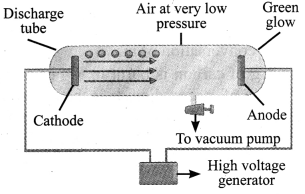→ At the ends of the discharge tube, two electrodes were placed, connected to a battery for high voltage supply. The electrode connected to the negative end was known as cathode and that to the positive end as anode.

→ A stream of negatively charged particles was observed coming out of the cathode towards the anode. These particles were called electrons. The collection of negatively charged particles emitted from the cathode in the discharge tube are called cathode rays.→ Proton (p+): In 1886, Goldstein observed in the same experiment, with different situation that, anode emitted positive particles which were called protons.

→ Canal rays: The positively charged radiations produced in the discharge tube from anode are called canal rays.

→ Difference between electron and proton:

 Electron Proton Its mass is negligible. Its mass is 1 unit. Charge is negative. Charge is positive. J.J Thomson discovered it. E. Goldstein discovered it.

→ Neutron (n): These neutrally charged particles were discovered by James Chadwick in 1932. Neutrons are present in atoms of all elements except hydrogen.

→ The structure of an atom:
1. Thomson’s Model of an atom: An atom is a positively charged sphere and the electrons are embedded in it. The magnitude of positive and negative charge is same inside an atom, so the atom is electrically neutral.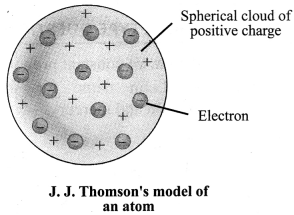2. Rutherford’s α-particle scattering experiment’: α-particles are doubly charged helium ions. Since they have a mass of 4 u, the fast moving α-particles have a considerable amount of energy. A thin gold foil with thickness of about 1000 atoms was taken. A lot of fast moving α-particles were bombarded on this thin gold foil. After passing through the foil, α-particles hit the screen.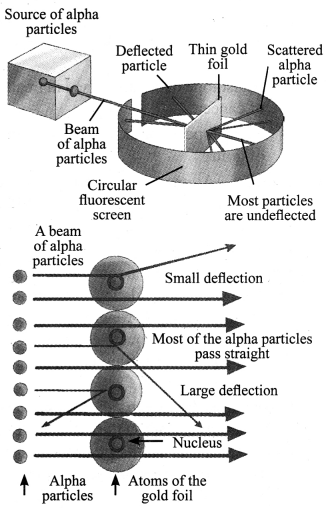Following are the observations and inferences made:

 Observation Inference a. Most of the α-particles pass through the foil without getting deflected. a. Most of the space inside the atom is empty. b. Some particles were deflected by small angles. b. Positive charge of the atom occupies very little space. c. One out of 12,000 particles rebound back. c. All the positive charge and mass of the gold atom were concentrated in a very small volume within the atom.

Rutherford, on the basis of this experiment, suggested ‘the nuclear model of an atom’. According to the nuclear model of an atom:
a. Most of the space inside the atom is empty.
b. At the centre of an atom, a small, heavy, positively charged nucleus is present.
c. Electrons revolve around the nucleus.
d. Total positive charge in nucleus is the same as total negative charge on all electrons of the atom as atom has net zero charge.→ Drawback of Rutherford’s model of an atom: If any charged particle undergoes accelerated motion, it must radiate energy. Therefore, if a charged body (e;) rotates around another charged body (nucleus), it will radiate energy. Due to energy loss through the radiation, speed of electrons will decrease and eventually jt will fall into the nucleus. But such collapse does not occur and atoms are found to be quite stable.

3. Bohr’s Model of Atom: In 1913, Neils Bohr overcame the limitations of Rutherford’s model and proposed a model of atomic structure.
Following are the postulates:
a. Electrons revolve around a centrally located heavy, small and positively charged nucleus in certain discrete orbits.
b. While revolving in discrete orbits, electrons do not radiate energy.
c. These discrete orbits or shells are called energy levels. These orbits or shells are represented by the letters K, L, M, N …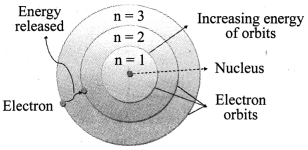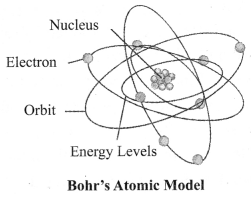→ Distribution of Electrons in Different Orbits: Distribution of electrons in different orbits (shells) is given by Bohr and Bury:
a. Maximum number of electrons present in a shell is given by 2n2 (n = shell number).
For example,
n = 1 (K shell). 2n2 = 2 (1)2 = 2 electrons
b. The maximum number of electrons that can be accommodated in the outermost orbit is 8.
c. Electrons are not accommodated in a given shell, unless the inner shells are completely filled.

→ Atomic Number: The number of protons present in the nucleus of an atom is called its atomic number. It is denoted by Z.

→ Mass Number: Mass number is equal to the number of nucleons present inside the nucleus of an atom. It means, it is the sum of the total number of protons and neutrons of an atom. It is denoted by the letter A.
‘Mass number of element = Number of protons + Number of neutrons’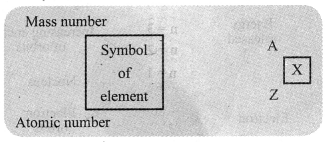→ Isotopes: Isotopes are atoms of same element having same number of protons but different number of neutrons. Isotopes have similar chemical properties but different physical properties.
For example: 126C, 146C

→ Uses of Isotopes:
a. An isotope of uranium (23592C) is used in nuclear power plants to generate electricity.
b. An isotope of cobalt is used in treatment of cancer.
c. An isotope of iodine is used in treatment of goitre.
Isobars: Atoms of different elements having same mass number but different atomic numbers are called isobars.
For example: 4020Ca, 4018Ar

→ Valency: The electrons in the last shell of the atom are called valence electrons. These govern the chemical properties of elements. The number of valence electrons that take part in a chemical reaction is called valency of the atoms.
For example, hydrogen has only one electron in its outermost orbit, thus it requires one more electron to fill its outermost orbit (K shell). For this, hydrogen atom shares one electron with another hydrogen atom and forms Hr

→ When an electron from a higher energy level falls in an orbit of lower energy level, the difference in energy is radiated in the form of electromagnetic radiation or light. This explains why different atoms on heating or under other conditions emit light of different colours.